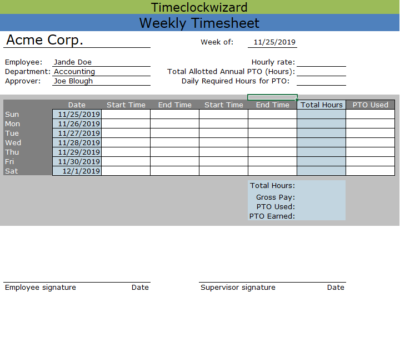# Weekly Timesheet Templates

## The Value of Weekly Timesheet Templates

Timesheet templates are a great way for managers to save valuable time and effort during their company’s payroll process. You probably already have a fair idea of how templates generally work, but the weekly timesheet template descriptions you’ll find on this page will help ensure that you’ll use them efficiently.

You’ll find several different kinds of weekly timesheet templates below. Time Clock Wizard also offers daily, bi-weekly, and monthly templates, but many companies are most comfortable about the weekly format we’ll discuss here. You’ll find more detailed descriptions of our weekly templates below, but let’s take a few moments to talk about the format in general.

Weekly timesheet templates can give you an up close look at your company payroll. While this definitely has value for some companies, weekly timesheets provide you with the wide angle view that many managers prefer. They’re also easier to fill out than daily timesheets, thus saving you and your people a significant amount of time.

## How the Templates Differ From One Another

As you’ll see in the descriptions below, there are a number of difference between our various weekly templates. For a complete account of these differences, it’s a good idea to consult the individual descriptions you’ll find below. But here’s a quick summary of how the templates might differ:

• Different templates cover different amounts of time– usually one or two weeks
• Some calculate overtime automatically while others don’t
• Sometimes, you’ll need to enter the start and end times of each employee, while other templates simply require the number of hours worked
• A few of these templates are capable of recording the work hours of multiple employees, but most can only deal with a single employee.

This list is not exhaustive– there are a few other differences between our various weekly time sheet templates– but it will be a great help in weighing your options.  In the following sections, you’ll find several different types of weekly timesheet templates. We’re quite certain that one will fit your needs perfectly.

### Contractor Weekly Timesheet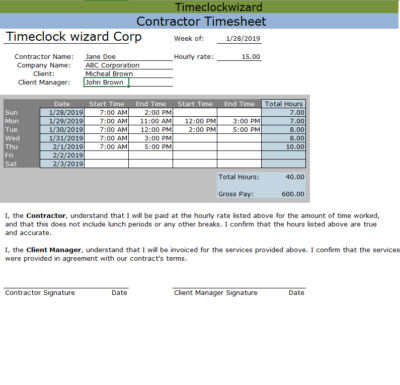• Includes space for two daily work periods (e.g. morning and afternoon).
• You enter the time of day that the employee starts and leaves work (eg 8:00 AM – 12:00 PM). The number of hours worked each day is calculated.
• The timesheet calculates overtime pay automatically.
• Areas with a blue background are automatically calculated in the spreadsheet version (dates, total hours, etc.)

### Simple Weekly Timesheet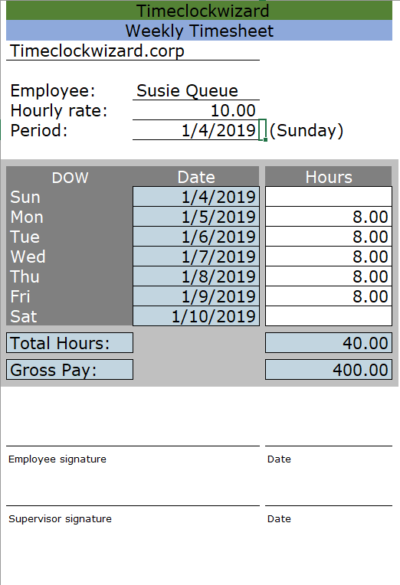• This is our most simple, no-frills type of timesheet.
• Spans one week. Calculations assume that the week starts on a Sunday.
• You enter the number of hours worked (e.g. 8.0) rather than hours being calculated based on start- and end-times.
• No calculation of overtime or comp time in this timesheet.
• Areas with a blue background are automatically calculated in the spreadsheet version (dates, total hours, etc.)

### Weekly Timesheet (horizontal orientation, work hours entered directly)• Spans one week. Calculations assume that the week starts on a Sunday.
• You enter the number of hours worked (e.g. 8.0) rather than hours being calculated based on start- and end-times.
• The timesheet calculates overtime pay automatically.
• Overtime is calculated on a daily basis; overtime rate is applied to hours in a given day that exceed the user-defined threshold for the day (e.g. over 8 hours).
• Days are oriented horizontally.
• Areas with a blue background are automatically calculated in the spreadsheet version (dates, total hours, etc.)

### Weekly Multiple-Employee Timesheet (work hours entered directly)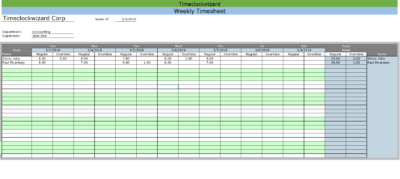• Tracks the workweek of multiple employees on one sheet.
• Spans one week. Calculations assume that the week starts on a Sunday.
• You enter the number of hours worked (e.g. 8.0) rather than hours being calculated based on start- and end-times.
• The timesheet calculates overtime pay automatically.
• Overtime is calculated on a daily basis; overtime rate is applied to hours in a given day that exceed the user-defined threshold for the day (e.g. over 8 hours).
• Days are oriented horizontally.
• Areas with a blue background are automatically calculated in the spreadsheet version (dates, total hours, etc.)

### Weekly Multiple-Employee Timesheet with overtime calculation, 1 work period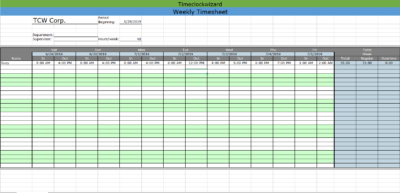• Tracks the workweek of multiple employees on one sheet.
• Spans one week. Calculations assume that the week starts on a Sunday.
• You enter the time of day that the employee starts and leaves work (eg 8:00 AM – 12:00 PM). The number of hours worked each day is calculated.
• The timesheet calculates overtime pay automatically.
• Overtime is calculated on a weekly basis; overtime rate is applied to hours in a given week that exceed the user-defined threshold for the week (e.g. over 40 hours).
• Days are oriented vertically.
• Areas with a blue background are automatically calculated in the spreadsheet version (dates, total hours, etc.)

### Self-Employed Weekly• You enter the number of hours worked (e.g. 8.0) rather than hours being calculated based on start- and end-times.
• No calculation of overtime or comp time in this timesheet.
• Areas with a blue background are automatically calculated in the spreadsheet version (dates, total hours, etc.)

### Weekly Timesheet (horizontal orientation)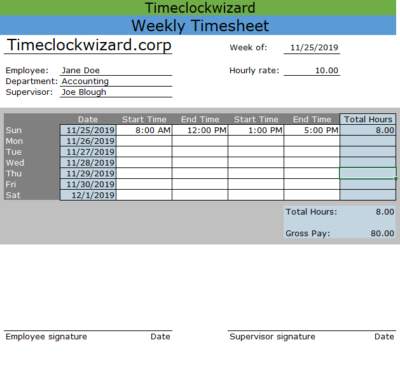• Spans one week. Calculations assume that the week starts on a Sunday.
• Includes space for two daily work periods (e.g. morning and afternoon).
• You enter the time of day that the employee starts and leaves work (eg 8:00 AM – 12:00 PM). The number of hours worked each day is calculated.
• No automatic calculation of overtime.
• Days are oriented horizontally.
• Areas with a blue background are automatically calculated in the spreadsheet version (dates, total hours, etc.)

### Weekly Payroll Sheet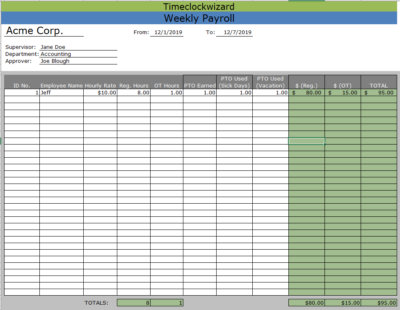• Spans 7 days.
• Includes spaces for up to 40 employees’ names, ID numbers, hourly rates, overtime rates, hours and PTO.
• End date, payment for employee regular hours and overtime, and total paid to all employees calculated automatically.
• User must fill in start date, hourly rates for both regular and overtime, and all hours worked.
• Areas with a green background are automatically calculated in the spreadsheet version.

### Weekly Projects Breakdown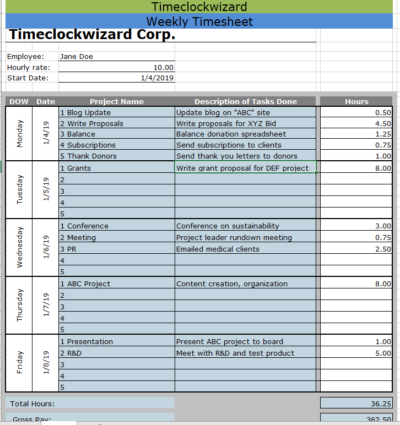• Includes space for two daily work periods (e.g. morning and afternoon).
• You enter the time of day that the employee starts and leaves work (eg 8:00 AM – 12:00 PM). The number of hours worked each day is calculated.
• The timesheet calculates overtime pay automatically.
• Areas with a blue background are automatically calculated in the spreadsheet version (dates, total hours, etc.)

### Weekly Timesheet With Daily PTO Calculation